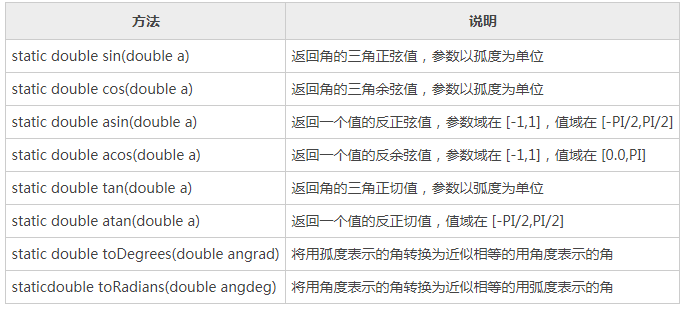# Java Math类的常用方法，三角函数运算

Math 类中包含的三角函数方法及其说明如下所示：```public class Test04
{
public static void main(String[] args)
{
System.out.println
{
"90 度的正弦值：" + Math.sin(Math.PI / 2));
System.out.println("0 度的余弦值：" + Math.cos(0));
System.out.println("1 的反正切值：" + Math.atan(l));
}
}```

```90 度的正弦值：1.0
0 的余弦值：1.0
1 的反正切值：0.7853981633974483
120 度的弧度值：2.0943951023931953```

Java Math类的常用方法，求整运算

Java Math类的常用方法，求最大值、最小值和绝对值

Java Math类的常用方法，静态常量